Next: Euler's method Up: Integration of ODEs Previous: Integration of ODEs

## Introduction

In this section, we shall discuss the standard numerical techniques used to integrate systems of ordinary differential equations (ODEs). We shall then employ these techniques to simulate the trajectories of various different types of baseball pitch.

By definition, an ordinary differential equation, or o.d.e., is a differential equation in which all dependent variables are functions of a single independent variable. Furthermore, an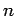th-order o.d.e. is such that, when it is reduced to its simplest form, the highest order derivative it contains isth-order.

According to Newton's laws of motion, the motion of any collection of rigid objects can be reduced to a set of second-order o.d.e.s. in which time,, is the common independent variable. For instance, the equations of motion of a set ofinteracting point objects moving in 1-dimension might take the form: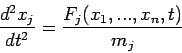(2)

forto, where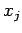is the position of the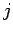th object,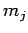is its mass, etc. Note that a set ofsecond-order o.d.e.s can always be rewritten as a set of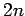first-order o.d.e.s. Thus, the above equations of motion can be rewritten: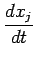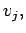(3)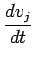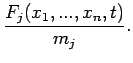(4)

forto. We conclude that a general knowledge of how to numerically solve a set of coupled first-order o.d.e.s would enable us to investigate the behaviour of a wide variety of interesting dynamical systems.Next: Euler's method Up: Integration of ODEs Previous: Integration of ODEs
Richard Fitzpatrick 2006-03-29